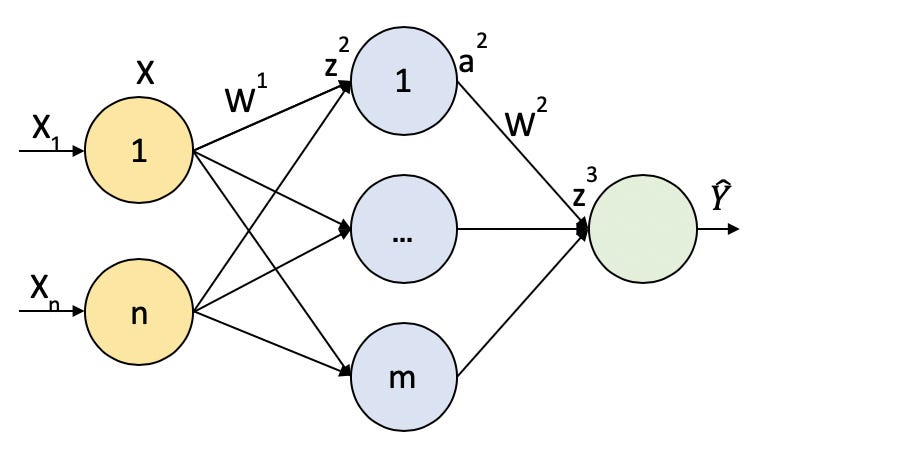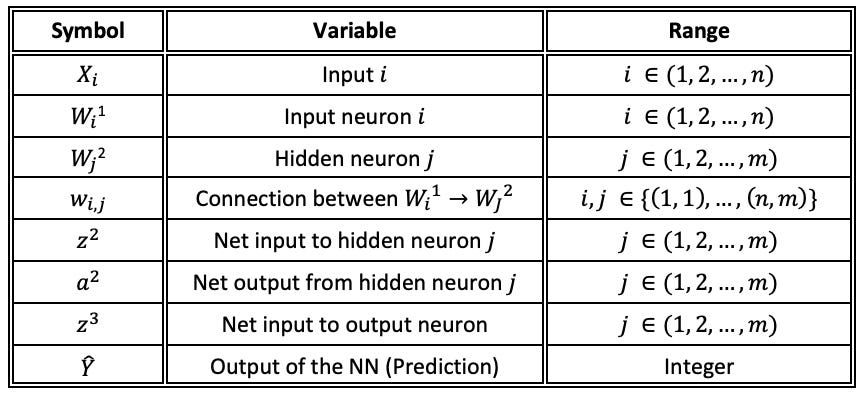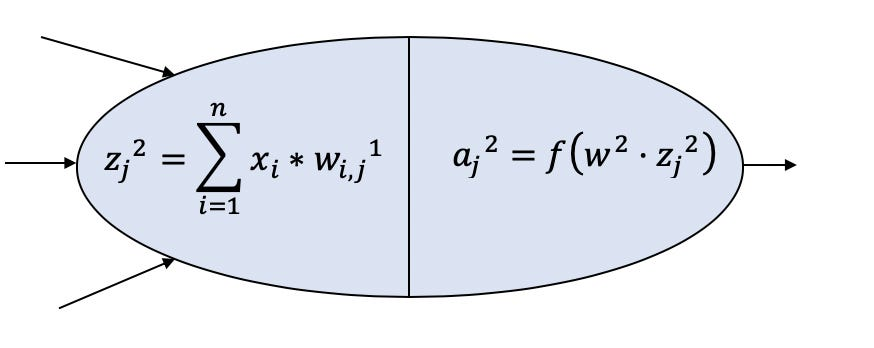# Neural Networks II: First Contact

Source: Deep Learning on Medium

This series of posts on Neural Networks are part of the collection of notes during the Facebook PyTorch Challenge, previous to the Deep Learning Nanodegree Program at Udacity.

### Contents

1. Introduction
2. Forward Pass
3. Backward Propagation
4. Learning
5. Testing
6. Conclusion

### 1. Introduction

In the next illustration, an Artificial Neural Network is displayed. We can see how we can forward our input data (X) into the NN and get a prediction for our output Y_hat.Figure 1. Schematic Feed Forward Neural Network

As we have the actual value that the prediction should have, we can compute the error using what is called a ‘Cost Function’. With these, we can see how responsible are each neuron for that error, and update their values in order to decrease that error with the time (actually, with the number of iterations over our input vector).

Just for easier visualization before writing down the equations, let’s move that NN to matrix notation:

Note that we are using n and m to refer that it could be any number, as well as it can also be more hidden layers. Just for simplification, we will go over the NN illustrated in Figure 1, which means 1 hidden layer, n=2, m=3.

In the next table, we can see also a table with the notation we are using in this document:Table 1. Notation used

### 2. Forward Pass

Now we have all this information, we can develop the mathematical approach over our NN. Just before it, also for easing the visualization, let’s see what is going on inside a neuron, for example our 1st hidden neuron.Figure 2. Single Neuron Scheme

This should remain the collector and distributor concepts introduced in Part 1 of this series.

According to the illustration, the neuron is adding all the inputs multiplied by their corresponding weight, and then applied an activation function to give the output to the next step.

The whole set of equation describing the NN is therefore:

With these equations, we complete the forward method that we will define in our Neural_Network class (we will start introducing Python notation also to be more familiar when looking at the code).

The Cost Function then, will be computed using that output of the NN and the real value (note this is supervised learning). There are several ways of computing this function, depending on your problem.

This time, we will try to minimize the RMSE (Root Mean Squared Error), which correspond with the next formula. RMSE is a typical error function used in regression problems as Cross-Entropy is for the classification problems. However, in both cases there is a variety of different functions that we could used

More interestingly, we could express the same equation depending on the parameters of the network:

### 3. Backward Propagation

It is the moment to tell the NN the error with respect to what the value shoud have bee and propagate it backwards to ‘tell’ the weights how much responsible they are for that error, so they can update their owns values in order to continuously decrease that error.

Thus, we want to know how much is the value of dJ/dW1 and dJ/dW2.

If we first develop the gradient correspond to the hidden layer weights:

Now, if we forget for a second about the constant term, by applying the chain rule to the remaining derivative term:

1–The prediction Y_hat is a function of z3, so it can be directly derived.

2–The prediction Y_hat can be plot against W2 as a straight line with slope a2. Mathematically, this is represented as the transpose of that vector. Also, we have grouped the remaining terms into the delta3, which is called the ‘Back Propagated Error’.

The above statements can be also revisited in this great youtube explanation.

And, now applying again the chain rule to next equation, we can compute the remaining gradient:

1–The input z3 can be plot against a2 as a straight line with slope w2 → w2.T

2–The input a2 is a function of z2, so it can be directly derived

3–The input z3 can be plot against a2 as a straight line with slope X → X.T

### 4. Learning — Weights update

So far, we have explained how the forward step is done to get a prediction, then how to use the real values to calculate the value of the Cost Function, and how using back-propagation (partial derivatives + chain rule) we can tell each neuron how responsible they are for that error. But now, how does our NN learn? Well, the same thing as we do: try, fail, learn. These steps are what we call: to train.

Thus, we have covered the try (forward) and the fail (Cost) steps. Now, the step left, learn, is accomplished by updating the value of the weights. Each node (or neuron) is going to update its last value, following the next equation:

That parameter lambda is called the ‘Learning Rate’. Thus, as dJ/dWi is the error J committed on the responsibility of Wi — (note Wi is an entire layer of weights) — . We are exactly telling our neurons to correct their own value given the mistake committed (by their fault) multiplied by this learning rate.

If the value of the learning rate is too high (usually start with 0.01 or 0.001), the processes of learning could not converge, and if the value is too low, it can take forever. Just like in real life! We want to learn as fast as possible, but we know that we need to go slow in the beginning to assimilate all the information we didn’t know, until we feel confident to go on and try with new information

(*) Eq. 9 is the simplest implementation of the learning. Normally, we say that an optimizer is the responsible to perform this update on the weights. There are many different implementations of optimizers. This case, the most basic and the pillar for the rest of them is known as Stochastic Gradient Descent. Here is a great post on different optimizers and how to implement them.

### 5. Testing — Have we learnt?

The process of learning is repeated over and over until we consider we have learnt. But, what does ‘have learnt’ mean? Just like studying for an exam, we usually do all the problems in the book collection, until we ensure that we are able to solve all of them. But then the exam comes. In the exam, there is (usually) data that you have not worked with, you don’t know what you are going to find!

But, if you have studied enough, you are pretty sure that if you apply you forward( ) function to the exam data, you will get an A+ with the outputs you’ll get (your answers). The behavior of NN are exactly the same. It trains until it is confident to forward new data. (Be careful with overfitting, training too much).

### 5. Conclusion — Have we learnt?

I hope this has been fun! This is just the start of the Neural Networks world! Now you can go to the GitHub repo to see how to code this from scratch or how it can be implemented using a deep learning framework like PyTorch.

I highly recommend you start programming from scratch and make sure you understand everything. Then you will be ready to save time using the libraries, but with the certain that you understand what is going on under the hood.

Anything you need, just leave the comment, and if you liked it, please recommend it! Until next time, enjoy Neural Networks!!